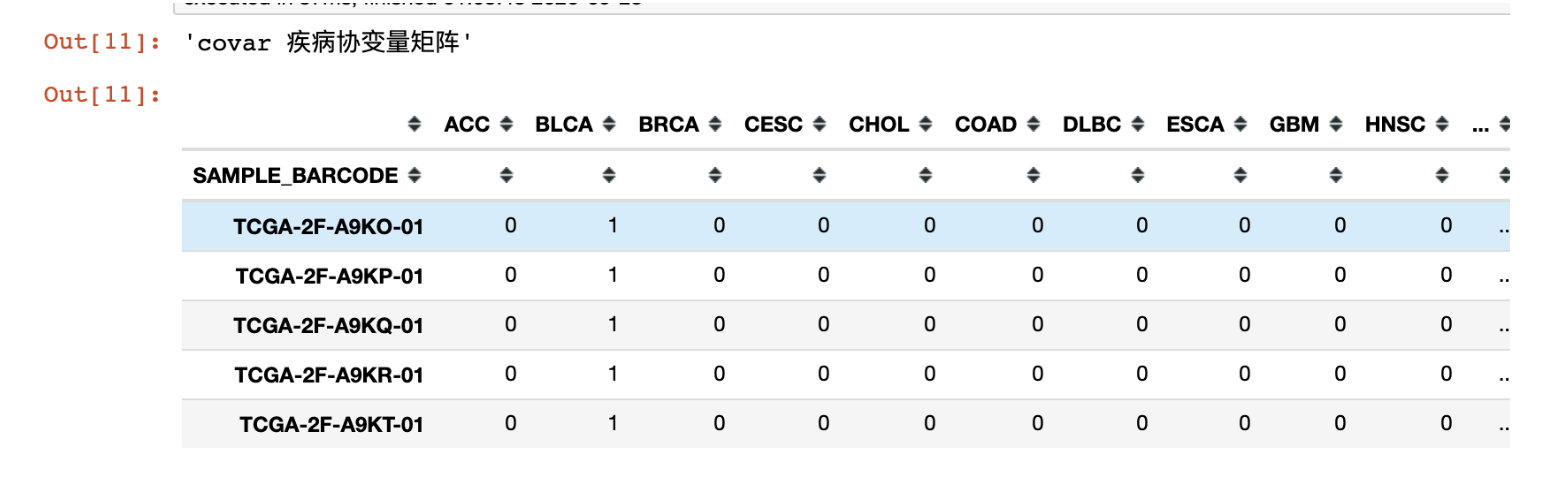# TCGA官方数据挖掘文章教你机器学习

### 还是深度学习香

深度学习可能大家都不陌生，不过我感觉大部分的人只停留在不陌生的阶段。虽然大家可能都知道，深度学习的主流应用方向还是图像识别，人脸识别，seq2seq ，或者目前非常火热的自动驾驶等等。这样子的话听起来似乎深度学习和生信一点关系都没有。

但是事实并非如此。到目前来说其实已经有很多的文章已经把深度学习的方法应用的计算生物学和生物信息学的领域之中了（这篇文章就是关于深度学习在生物信息学领域应用的综述），所以本次系列课程的任务重心旨在把深度学习的各种方法合理的应用在生物信息学的各个领域之中(尤其是各组学领域，但是因为我对基因组的领域不是很熟悉，所以重心可能会放在下游的表达谱这一块)

### Why Deep learning?

为什么选择深度学习而不是机器学习，最重要的原因就是上面提到的鲍志炜师兄已经做过了，我觉得我做肯定不如他，所以就直接跳到深度学习了。

第二个原因就是，深度学习所需要的大数据刚好在生信领域完美契合，有很多公共的大型数据库供我们使用，最典型的就是 TCGA、GEO这两个我们非常熟悉的数据库

并且我们这次系列主要还是以实战为主，头篇参考的文章是来自 TCGA 官方的一篇文章Machine Learning Detects Pan-cancer Ras Pathway Activation in The Cancer Genome Atlas30389-9)文章的源码也可以在官方的 github 上搜到。虽然这篇文章我在我的 github 上发过一次源码教程（在生信菜鸟团也发过一部分，但是由于审核的问题后面就没发了）。但是这一次系列课程是完全不一样的，因为 TCGA 用的是机器学习的方法，我打算使用深度学习的方法重启这篇文章

python 相关的语法也是不会有任何的讲解的，万能的B站太多视频资料了，所以默认大家都会python哈！

### 万物皆函数！

OK，不讲废话了，直接进入系列的第一个篇章。这篇文章的目的很明确——利用基因表达谱+额外的信息从而预测癌样本 RAS 通路激活情况。在多说一句废话：由于 TCGA 上可以利用的资源比较多，也可以尝试用同样的思路预测别想要的信息

那么，深度学习到底学习的是什么呢？下面直接用文献中的图来解释：这张图就基本上解释了机器学习或者深度学习的本质——函数映射。对，就是那个我们在中学的时候就学过的函数 `f(x) = y` ，这个函数的映射关系可以用到宇宙万物。

例如，我们可以将 x 记录为：

1. 早晨起来投的硬币是正面还是反面
2. 今天天气怎么样
3. 出门穿的是什么样的衣服
4. …..

预测：y 为晚餐吃得是什么？

或许有的人认为这个是扯淡的预测，但是很遗憾，并不是，因为只要我们能够找到正确的 `f(x)—>y` 的映射关系，就一定能够根据 x 的信息预测这个人晚餐吃得是啥，因为马克思说过：联系是事务普遍的规律。之所以觉得不可能，是因为光靠我们这个脑子是无法计算出一个正确的映射关系的。因此，对于任何一个机器学习还是深度学习，其本质就是找到一个映射关系将 x 映射到 y。当然，实际情况及其复杂一般都是这样的映射网络 `f1(f2(f3(f4(f5(.....fn(X))))))` 就和俄罗斯套娃一样套了好几层的映射关系。因此在很多情况下，即便是机器都很难学习到真正的映射网络。在实际情况中，学习的到的映射模型只是一种和真实模型很像的模型。但即便是这样，这种和很像的模型通常情况下也能够解决很多事情。

### 学习的过程？

接下来的问题就在于要怎么让电脑去学习这个映射关系 `f()`或者说`f1(f2(f3(f4(f5(.....fn(X))))))`，我们从最简单的模型开始讲吧!机器的学习过程和人的学习过程其实非常像，回顾一下我们备考四六级的时候的样子我们每天埋头备考，我们买了一沓的星火英语，华研外语等等，里面有模拟题`（准备训练集样本）`也有答案`（准备训练集标签）`,然后开始做题`（f(.)）`，做完核对，发现做错了几十道题目了甩自己几十耳光`（计算loss）`，不懂的找知识点`（优化器）`，然后回去查看一下到底为什么做错`（反向传递）`，然后继续做题`（修正f(.)）`。然后继续做题继续错继续抽自己继续找知识点。。。。`（迭代）`。然后上考场`（验证集）`最后考了666 分。这样就训练出了一个较为完美深度学习框架。

总结一下到底怎样四六级可以考高分呢`（训练一个好的模型）`？首先是肯定要有模拟题`(训练集)`，模拟题越多越好，当然这里又要关心模拟题的质量，如果你拿少儿剑桥英语去备考四六级`（质量低的数据）`，那么即便你做再多的题目也没用，此外模拟题的答案也很重要`（训练集标签）`，如果你的答案就是错的，那么在核对的过程你只会加深错误的印象，考试就不可能考高分`（模型泛化能力差）`。那么题目要怎么做呢？`f(.)（隐藏层的设置）`我们要针对四六级的题型去选择题型去做`（隐藏层类型）`，每种题型做几道`(隐藏层个数)`，这些都会影响到我们最后考试的成绩。除此之外合理的惩罚制度`（loss 函数的选择）`也非常重要，错 100 题都不愿意甩自己一巴掌的人最后考试的结果肯定不如错一题甩自己一巴掌的人考的好（当然，如果没做错也乱甩自己到最后自己都不知道自己哪里错了也不行）。最后，如何整理和找到自己真正不懂的知识点也及其的重要`（优化器的选择）`，这一点如果做得好，会让你事半功倍

综上，机器学习最重要的组件有：

1. 较为良好的数据和相对正确的标签（这一点在生信领域尤为重要，因为图像识别领域标签一定是对的，但是我们这里的话定义就很模糊，后期会讲）
2. 隐藏层的设置
3. loss 函数的选择
4. 优化器

### final

那么，回到我们生信相关的具体的例子来说就是，x 就是患者所有基因的表达值，Y 就是想要预测的特征（某个基因的突变，患者生存风险，某条通路的异常等等），接下来的教程我会直接用 TCGA 的数据进行实战演练，带领大家做完一次完整的深度学习框架

### 建议

1. 如果可以的话，希望有兴趣和我一起学习深度学习的小伙伴们可以吧上面 TCGA 官方的文章的内容复现一遍。
2. 如果不是数学或者物理科班出生（或者说高中以来没有参加过数学竞赛的，考研数一没 135 的）的然后转行到生信的建议在后续的教程中关于一些数学知识不懂的话没有必要死磕，因为并不影响调参
3. 如果有不信邪的可以试着尝试先看一下 B 站的一个白板系列劝退课程

### 第二篇笔记

没有意义的开场的废话就不讲了，直接先上手跑一遍大概的流程

（接上一篇的 TCGA 文章）原始数据我们就不用了，直接用他处理好的数据：https://github.com/greenelab/pancancer### Start

``````# 导入模块
import os
import math
import torch # pythorch 模块
import itertools
import warnings

import numpy as np
import pandas as pd
import matplotlib.pyplot as plt

from torch import nn

T = True
F = False
%matplotlib inline
%config InlineBackend.figure_format = 'svg'

warnings.filterwarnings("ignore")
plt.rc('font', family='Times New Roman')
plt.rcParams['figure.figsize'] = (4, 3)
my_colors = ["#1EB2A6", "#ffc4a3", "#e2979c", "#F67575"]
``````

接下来呢我们就按照文章的代码进行数据预处理，当然，只有数据预处理的这一部分是一样的，后续的建模过程会完全不一样

``````# 数据导入

# 查看数据类型
"""sample_freeze 样本肿瘤信息矩阵"""
sample_freeze.shape
"""mut_burden 样本肿瘤突变负荷"""
mut_burden.shape
"""rnaseq_full_df rnaseq表达谱"""
rnaseq_full_df.shape
"""mutation_df mutation(0-1)矩阵"""
mutation_df.shape
``````接下来就是提取文章中要预测的RAS 通路相关的信息

``````genes = 'KRAS,HRAS,NRAS'
genes = genes.split(',')
common_genes = set(mutation_df.columns).intersection(genes) # 目标基因和mutation矩阵基因交集
common_genes = list(common_genes.intersection(rnaseq_full_df.columns)) # 交集后和rnaseq矩阵交集
y = mutation_df[common_genes] # 提取目标基因中和mutation矩阵和rnaseq交集的基因,在样本中的突变情况
"""y"""
``````不要忘记把表达谱中如果有测到这个基因的话是要删除的，不然的话后续的预测就没有意义了

``````print("移除的基因是 : %s" %common_genes )
rnaseq_full_df.drop(common_genes, axis=1, inplace=True)
# 移除的基因是 : ['HRAS', 'NRAS', 'KRAS']
``````

1. 提取突变信息（上面已经提取过）
2. 提取基因的拷贝数变异信息
3. 如果是抑癌基因，则考虑是否有拷贝数删失，如果是原癌基因则考虑是否有拷贝数增加
4. 整合拷贝数变异信息和突变信息，只要有一个突变则认为样本 RAS 通路异常

``````loss_df = pd.read_table('data/copy_number_loss_status.tsv.gz', index_col=0) # 缺失
'''癌基因分类表'''
cancer_genes_df[['Gene Symbol','Ocogene score* ','Tumor Suppressor Gene score* ']].iloc[0:5,:]
genes_sub = cancer_genes_df[cancer_genes_df['Gene Symbol'].isin(common_genes)]# 获取基因信息

tumor_suppressor = genes_sub[genes_sub['Classification*'] == 'TSG'] # 提取抑癌基因
oncogene = genes_sub[genes_sub['Classification*'] == 'Oncogene'] # 提取原癌基因
copy_loss_sub = loss_df[tumor_suppressor['Gene Symbol']] # 如果是抑癌基因则获取拷贝数缺失信息
copy_gain_sub = gain_df[oncogene['Gene Symbol']] # 如果是原癌基因则获取拷贝数增加信息

copy_loss_sub.columns = [col + '_loss' for col in copy_loss_sub.columns] # 修改列名
copy_gain_sub.columns = [col + '_gain' for col in copy_gain_sub.columns]

# Add columns to y matrix
y = y.join(copy_loss_sub) # 合并拷贝数变异信息
y = y.join(copy_gain_sub)

# 数据清洗
y = y.fillna(0)
y = y.astype(int)
# 三个目标基因中只要有一种基因突变则人为患者为突变型
y['total_status'] = y.max(axis=1)
y = y.reset_index().merge(sample_freeze,
how='left').set_index('SAMPLE_BARCODE') # 合并样本疾病类型
'''y'''

count_df = y.groupby('DISEASE').sum() # 计算不同癌的型基因突变频率
prop_df = count_df.divide(y['DISEASE'].value_counts(sort=False).sort_index(),
axis=0)

count_table = count_df.merge(prop_df, left_index=True, right_index=True,
suffixes=('_count', '_proportion'))
count_table.to_csv("count_table_file.csv")
``````

移除肿瘤突变负荷过高的样本

``````ras_diseases='BLCA,CESC,COAD,ESCA,HNSC,LUAD,LUSC,OV,PAAD,PCPG,READ,SKCM,STAD,TGCT,THCA,UCEC'
diseases = ras_diseases
diseases = diseases.split(',')
y_df = y[y.DISEASE.isin(diseases)].total_status # 提取指定疾病的基因患者突变信息
common_samples = list(set(y_df.index) & set(rnaseq_full_df.index)) # 提取指定疾病的基因表达谱
y_df = y_df.loc[common_samples]
rnaseq_df = rnaseq_full_df.loc[y_df.index, :]
burden_filter = mut_burden['log10_mut'] < 5 * mut_burden['log10_mut'].std()
mut_burden = mut_burden[burden_filter]

y_matrix = mut_burden.merge(pd.DataFrame(y_df), right_index=True,
left_on='SAMPLE_BARCODE').set_index('SAMPLE_BARCODE')
``````

添加协变量信息

``````y_sub = y.loc[y_matrix.index]['DISEASE']
covar_dummy = pd.get_dummies(sample_freeze['DISEASE']).astype(int)
covar_dummy.index = sample_freeze['SAMPLE_BARCODE']
covar = covar_dummy.merge(y_matrix, right_index=True, left_index=True)
covar = covar.drop('total_status', axis=1)

'''covar 疾病协变量矩阵'''
"""y_sub 样本肿瘤信息"""
"""y_df 目标基因患者突变状态"""
````````````y_df = y_df.loc[y_sub.index] # 基因突变矩阵
strat = y_sub.str.cat(y_df.astype(str)) # 疾病类型
x_df = rnaseq_df.loc[y_df.index, :] # 基因表达矩阵
'''疾病类型+0/1'''
'''例如: CESC0'''
'''表示患者属于cesc 且目标基因未突变'''
from sklearn.preprocessing import StandardScaler
fitted_scaler = StandardScaler().fit(x_df)
x_df_update = pd.DataFrame(fitted_scaler.transform(x_df),
columns=x_df.columns)
x_df_update.index = x_df.index
x_df = x_df_update.merge(covar, left_index=True, right_index=True)
x_df.shape
x_df.to_csv("raw_exp.csv")
y_df.to_csv("raw_clinic.csv")
y_sub.to_csv("strat.csv")
``````

### 正文

关于深度学习的内容，我会以 pytorch 为基本开始展开这个系列当然官方也给出了教科书，不过是纯英语的（该练英语了）包括在网上也有很多入门教程，所以我这个系列的定位是实战，虽然网上也有很多实战的内容，但是我这个系列是主要在表达谱这个版块的应用不要总是天天 limma,deseq2,edgr，时代变了赶紧跟着我的系列进入新时代。

1. 较为良好的数据和相对正确的标签
2. 隐藏层的设置
3. loss 函数的选择
4. 优化器

### Start

``````import os
import math
import torch # pythorch 模块
import itertools
import warnings

import numpy as np
import pandas as pd
import matplotlib.pyplot as plt
import torch.utils.data as Data
from torch import nn
from sklearn.model_selection import train_test_split
from sklearn.metrics import roc_curve,precision_recall_curve ,roc_auc_score,auc,average_precision_score

T = True
F = False
%matplotlib inline
%config InlineBackend.figure_format = 'svg'

warnings.filterwarnings("ignore")
plt.rc('font', family='Times New Roman')
plt.rcParams['figure.figsize'] = (4, 3)
my_colors = ["#1EB2A6", "#ffc4a3", "#e2979c", "#F67575"]
def roc_plot(label=None,scores=None,title=None,file_name=None, save=False):
fpr, tpr, threshold = roc_curve(label, scores) ###计算真正率和假正率
roc_auc = roc_auc_score(label, scores) ###计算auc的值
plt.figure(figsize=figsize)
plt.plot(fpr, tpr, color=my_colors, lw=lw,
label='AUC: %0.4f' % roc_auc) ###假正率为横坐标，真正率为纵坐标做曲线
plt.plot([0, 1], [0, 1], color=my_colors, lw=lw, linestyle='--')
plt.xlim([-0.01, 1.01])
plt.ylim([-0.01, 1.01])
plt.xlabel('1 - Sepcificity', size=label_size)
plt.ylabel('Sensitivity', size=label_size)
plt.yticks(fontsize=ticks_size)
plt.xticks(fontsize=ticks_size)
plt.title(title, size=title_size)
#plt.grid(True)
plt.legend(bbox_to_anchor=legend_sit,
fontsize=legend_size,
if save:
plt.savefig(file_name)
plt.show()
plt.clf()
``````

把上一次的数据导入

``````Expr = pd.read_csv('raw' + '_exp.csv', index_col=0) # 表达谱
Clinic = pd.read_csv( 'raw' + '_clinic.csv', index_col=0) # label
strat = pd.read_csv('strat.csv', index_col=0) # 分层信息
strat = strat['DISEASE'].str.cat(Clinic.astype(str))

``````

拆分样本

``````Samples = Expr.index # 样本名
Genes = Clinic.columns # 基因名
Expr = np.array(Expr) # 转换为 numpy.array
Clinic = np.array(Clinic["total_status"]) # 这里注意下，因为我们导入的形式是data.frame的虽然只有一列，但是如果直接转为 numpy 会出现问题，你们可以尝试一下
x_train, x_test, y_train, y_test = train_test_split(
Expr,
Clinic,
test_size=0.1, # 验证集比例
random_state=0, # 随机种子
stratify=strat #分层抽样
) # 这里用到了 sklearn 的库

del Expr, Clinic ## 删除变量释放一下内存，不然会卡死

# 将数据转为 torch
x_train = torch.from_numpy(x_train)
x_test = torch.from_numpy(x_test)
y_train = torch.from_numpy(y_train)
y_test = torch.from_numpy(y_test)

x_train = x_train.float()
x_test = x_test.float()
# 将 label 转为 tensor 类型
y_train = torch.LongTensor(y_train)
y_test = torch.LongTensor(y_test)
``````
``````batch_size = 512
# 将训练集分割成小 batch，当然也可以不分割，但是我劝你最好还是做，当然你也可以不听劝
train_iter = Data.TensorDataset(x_train, y_train)
dataset=train_iter, # 数据，封装进Data.TensorDataset()类的数据
batch_size=batch_size, # 每块的大小
shuffle=False, # 要不要打乱数据 (这里不用打乱顺序)
num_workers=8, # 多进程（multiprocess）来读数据
)

# 验证集不分割
test_iter = Data.TensorDataset(x_test, y_test)
dataset=test_iter, # 数据，封装进Data.TensorDataset()类的数据
batch_size=x_test.shape, # 每块的大小
shuffle=False, # 要不要打乱数据
num_workers=8, # 多进程（multiprocess）来读数据
)
``````

构建神经网络

``````# 组件 2 隐藏的设置
# 这里我们定义一个最最最最最简单的网络
class MyNet(nn.Module):
def __init__(self):
super(MyNet, self).__init__()
self.mlp = nn.Sequential(nn.Linear(x_train.shape, 512), nn.ReLU(),
nn.Linear(512, 256), nn.ReLU(),
nn.Linear(256, 2), nn.Sigmoid())
def forward(self, x):
return self.mlp(x)

Net = MyNet()
def Trainning(Net=None,
train_iter=None,
test=None,
loss=None,
num_epochs=None,
optimizer=None):
for epoch in range(num_epochs):
train_loss, train_acc,n = 0.0,0.0,0
for X,y in train_iter:
y_pred = Net(X)
l = loss(y_pred,y).sum()

l.backward()
optimizer.step()

train_loss += l.item()
train_acc += (y_pred.argmax(dim=1) == y).sum().item()
n += y.shape
for test_X ,test_y in test_iter:
test_acc = (Net(test_X).argmax(dim=1) == test_y).sum().item()/len(y_test)
print('epoch %d, loss %.4f, train acc %.3f, test acc %.3f'
% (epoch + 1, train_loss / n, train_acc / n, test_acc))
``````

训练模型

``````# 组件 3loss
# 选择分类交叉熵作为损失函数
loss = nn.CrossEntropyLoss()
num_epochs = 5 # 先训练 5 轮看看
# 组件 4 优化器
Trainning(Net,
train_iter,
test_iter,
loss,
num_epochs,
optimizer)
``````AUC

``````with torch.no_grad():
prob= Net(x_test).numpy()
pridict = Net(x_test).argmax(dim=1)
scores = [i for i in prob]
label = y_test

# 定义函数
lw = 3.5
ticks_size = 12
label_size = 16
title_size = 18
legend_size = 14
legend_sit = (0.85, 0.3)
figsize = (5, 5)
roc_plot(label,
scores,
title="validation"
#file_name='AUC.pdf',
#save=True)
)
``````

###Final

不知道看完大家有什么感受，可能是云里雾里一脸懵逼（虽然我觉得这可能是我教程写的有点太差了，这一点我会尽可能的改进，所以不要喷，毕竟写系列教程我也是第一次）。代码这种东西确实比较枯燥，我也想在别写代码的时候尝试添加一下自己的话，这样有点互动的感觉，而不是整片文章都是代码，因为我最近事情比较多，所以学习的过程可能比较就，但是我也有抽时间把老师写的 R 语言入门教程看了一下，模仿一下老师学习如何写一个比较优秀的入门教程

希望之后的教程能写好一点吧。。。